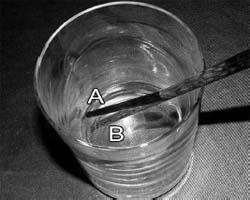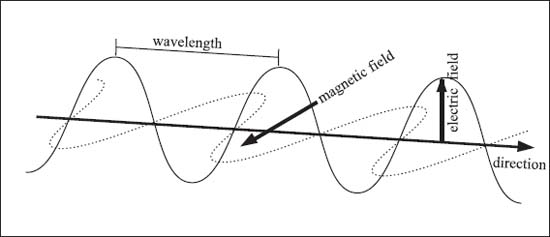NextPrevious

# Is the speed of light always the same?

The speed of light actually depends on what material the light is moving through! Each material has a property called an index of refraction. From the index of refraction, we can calculate the speed of light passing through a material from the following equation:

In this equation, c is the speed of light in a vacuum (about 2.998 × 108 m/s), n is the index of refraction of the material in question, and ν tells us the velocity of light in the material.Electromagnetic radiation consists of electric and magnetic fields that run perpendicular to one another. The wavelength of light is the distance measured between wave crests.

Close

This is a web preview of the "The Handy Chemistry Answer Book" app. Many features only work on your mobile device. If you like what you see, we hope you will consider buying. Get the App